# RBSE Maths Class 10 Chapter 13: Circle And Tangent Important Questions and Solutions

RBSE Class 10 Maths Chapter 13 – Circle and Tangent Important questions and solutions are provided here. All these questions are given by our expert faculty to help the students in clearing their exams with flying colours. The RBSE Class 10 important questions and solutions given at BYJU’S has a full coverage of all the concepts of Class 10.

Chapter 13 of RBSE Class 10 contains only two exercises, which involve questions pertaining to the relation between tangents and other parts of the circle. Students will be introduced with different types of questions such as finding the radius of the circle when the length of the tangent and distance of a point from which the tangent is drawn to the centre of the circle.

### RBSE Maths Chapter 13: Exercise 13.1 Textbook Important Questions and Solutions

Question 1: Write true or false. Also, write the reason for your answer.

(i) Tangent of a circle is the line which intersects the circle at two points.

(ii) A tangent XY, touches a circle at point P whose centre is O and Q is any point on the tangent, then OP = OQ.

(iii) LM and XY are two tangents at the points P and Q on the circle. If PQ is diameter, then LM ∥ XY.

(iv) O is the centre of a circle situated on the other circle whose centre is A. If a circle having centre O passes from A and B such that AOB are in one line, then the tangents drawn from B will pass through the intersecting points of both circles.

Solution:

(i) False – Tangent intersects the circle at one and only one point.

(ii) False – OP is perpendicular to the tangent and perpendicular is the smallest among the distances.

(iii) True – Tangent is perpendicular to its diameter.

(iv) True – AOB is a diameter and the angle in a semicircle is always a right angle.

Question 2: The length of tangent is 4 cm, drawn from any point which is 10 cm away from the centre of the circle. What will be the radius of that circle?

Solution:

Let PQ be the tangent to the circle with centre O, at point P on it.

PQ = 4 cm

OQ = 10 cm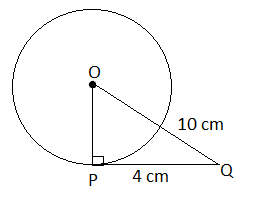We know that the tangent drawn at any point on the circle is perpendicular to the radius at the point of contact.

Therefore, ∠OPQ = 90°

In right triangle OPQ,

OQ2 = PQ2 + OP2

102 = 42 + OP2

OP2 = 102 – 42

= 100 – 16

= 84

OP = √84 cm

OP = 2√21 cm

Hence, the measure of radius of the circle is 2√21 cm.

Question 3: A circle with centre at O touches the four sides of a quadrilateral ABCD internally in such a way that it divides AB in 3 : 1 ratio. If AB = 8 cm, then find the radius of the circle where OA = 10 cm.

Solution:

Given,

A circle with centre O touches the four sides of a quadrilateral ABCD of the point E, F, G and H respectively.

Let E divides the side AB in the ratio 3 : 1.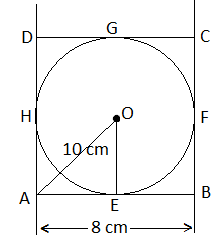OA = 10 cm

AB = 8 cm

Let AE = 3x

EB = x

∴ AB = AE + EB

⇒ 8 = 3x + x

⇒ 4x = 8

⇒ x = 8/4 = 2 cm

AE = 3 × 2 = 6 cm

∴ EB = x = 2 cm

In right triangle AEO,

OA2 = AE2 + OE2

(10)2 = (6)2 + (OE)2

OE2 = (10)2 – (6)2

= 100 – 36

= 64

OE = √64

OE = 8 cm

Hence, the radius of the circle is 8 cm.

Question 4: In the figure, the centre of a circle is O and the tangents drawn from a point P are PA and PB which touches the circle at A and B respectively, then prove that OP is the bisector of line AB.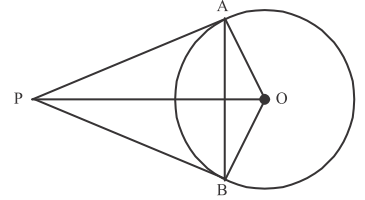Solution:

Let OP intersects the chord AB at C.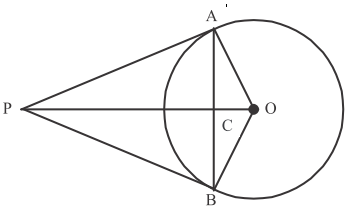In ΔPCA and ΔPCB,

PA = PB (tangents to the circle from external point P)

PC = PC (common)

∠APC = ∠BPC (tangents PA and PB, make equal angle with OP)

By SAS congruence criterion,

ΔPCA ≅ ΔPCB

⇒ AC = BC

Therefore, OP bisects line segment AB.

Hence proved.

Question 5: In the figure, O is the centre of the circle and from an exterior point P, two tangents PA and PB are drawn to the circle at A and B respectively, then prove that PAOB is a cyclic quadrilateral.Solution:

Tangent at any point of a circle is perpendicular to the radius through the point of contact.

Thus, OA ⊥ PA and OB ⊥ PB.

∴ ∠OAP = ∠OBP = 90°

⇒ ∠OAP + ∠OBP = 90° + 90° = 180°

∠PAO + ∠AOB + ∠OBP + ∠BPA = 360°

⇒ ∠AOB + ∠BPA + 180° = 360°

⇒ ∠AOB + ∠BPA = 360° – 180°

⇒ ∠AOB + ∠BPA = 180°

That means, the sum of the opposite angles of the quadrilateral is 180°.

Hence proved.

### RBSE Maths Chapter 13: Exercise 13.2 Textbook Important Questions and Solutions

Question 6: According to the figure given below, answer the following questions.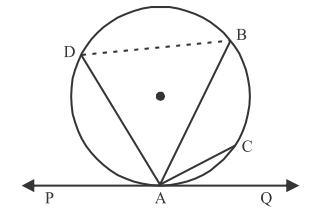(i) ∠BAQ is an alternate segment of the circle.

(ii) ∠DAP is an alternate segment of the circle.

(iii) If B is joined with C, then ∠ACB is equal to which angle?

(iv) ∠ABD and ∠ADB are equal to which angles?

Solution:

From the given figure,

(i) Alternate segment of ∠BAQ = ADB

(ii) Alternate segment of ∠DAP = ACBD

(iii) ∠ACB = ∠BAP (alternate angles)

(iv) ∠ABD = ∠DAP and ∠ADB = ∠BAQ

Question 7: In the figure, if ∠BAC = 80°, then find the value of ∠BCP.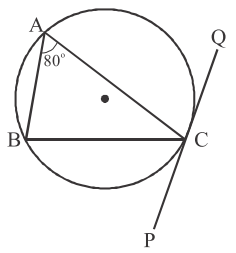Solution:

Given,

∠BAC = 80°

We know that, alternate segment of ∠BCP = ∠BAC

∠BCP = ∠BAC

⇒ ∠BCP = 80°

Question 8: In the figure, PQ and XY are parallel tangents. If ∠QRT = 30°, then find the value of ∠TSY.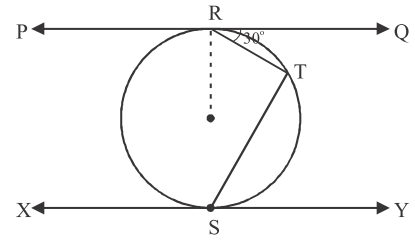Solution:

Given,

PQ ∥ XY

∠QRT = 30°

Let O be the centre of the circle join OS.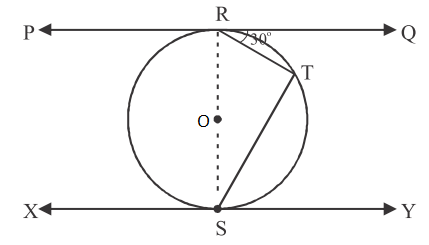RS is the diameter of the circle.

We know that tangent perpendicular to the radius of the circle through the point of contact.

∴ OR ⊥ PR and OS ⊥ XY

Thus, ∠QRO = 90°

⇒ ∠QRS = 90°

∠RTS = ∠QRS – ∠QRT

= 90° – 30° = 60°

∴ ∠TRS = 60°

∠TSY = ∠TRS (alternate segment)

⇒ ∠TSY = 60°

Question 9: In the figure, in a cyclic quadrilateral ABCD, diagonal AC bisects the angle C. Prove that diagonal BD is parallel to the tangent PQ of a circle which passes through the points A, B, C and D.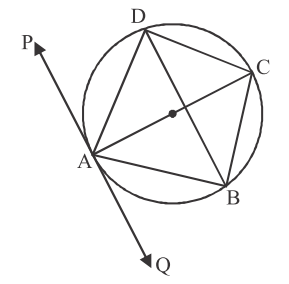Solution:

Given,

∠ACD = ∠ACB

Angles in the alternate segment are equal.

Similarly,

AB is a common arc, ∠ADB and ∠ACB are the angles in the same segment.

Similarly,

∠ABD = ∠ACD ….(iii)

From (i), (ii), and (iii),

⇒ PQ || BD

Hence proved.

### RBSE Maths Chapter 13: Additional Important Questions and Solutions

Question 1: Prove that if a chord is drawn from a point of contact of the tangent of the circle, then the angle made by this chord with the tangent are equal to the respective alternate angles made by segments with this chord.

Solution:

Let PQ be the tangent to the circle with centre O at point A.

Also, AB be the chord and C, D be the points of segments made by this chord and tangent.

∠1 and ∠2 are the angles made by the chord AB with the tangent PQ.

∠3 and ∠4 are the respective alternate angles of ∠1 and ∠2 made in the alternate segments at point C and D.

Join AE and BE.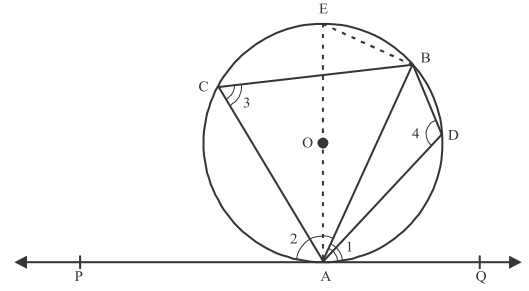In triangle AEB,

∠ABE = 90° (angle in the semicircle)

∠AEB + ∠EAB = 90° ….(i)

∠EAP = 90° (diameter and radius is perpendicular to the tangent through the point of contact)

∠EAB + ∠1 = 90° ….(ii)

From (i) and (ii),

∠EAB + ∠1 = ∠AEB + ∠EAB

∠1 = ∠AEB ….(iii)

We know that angles in the same segment are equal.

∠AEB = ∠3 ….(iv)

From (iii) and (iv),

∠1 = ∠3 ….(v)

∠1 + ∠2 = 180° (linear pair)

Sum of the opposite angles of a cyclic quadrilateral is 180°.

∠3 + ∠4 = 180°

Thus, ∠1 + ∠2 = ∠3 + ∠4

∠2 = ∠4 [From (i)]

Hence proved.

Question 2: From a point Q, the length of the tangent to a circle is 15 cm and the distance of Q from the centre of the circle is 17 cm, then find the radius of the circle.

Solution:

Let O be the centre of the circle and OP be its radius.

PQ be the tangent at point P and OQ be the distance from centre to point Q.

PQ = 15 cm

OQ = 17 cm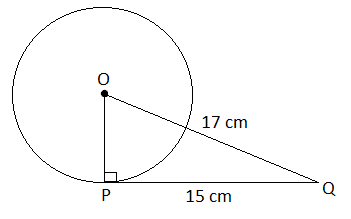In right triangle OPQ,

OQ2 = OP2 + PQ2

⇒ OP2 = OQ2 – PQ2

= (17)2 – (15)2

= 289 – 225

= 64

⇒ OP = √64

⇒ OP = 8 cm

Hence, the radius of the circle is 8 cm.

Question 3: Prove that the angle between the two tangents drawn from an external point to a circle is supplementary to the angle subtended by the line segment joining the points of contact at the centre.

Solution:

Let O be the centre and P be an external point from which two tangents PA and PB are drawn.

These two tangents touch the circle at point A and B, respectively.

AB is the line segment joining the points of contacts A and B such that it subtends ∠AOB at the centre of the circle.

Angle between two tangents = ∠APB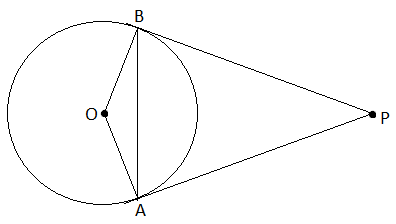We know that radius perpendicular to the tangent through the point of contact.

OA ⊥ PA

Thus, ∠OAP = 90°

Similarly,

OB ⊥ PB

Thus, ∠OBP = 90°

Sum of all the interior angles is 360°.

∠OAP + ∠APB + ∠PBO + ∠BOA = 360°

90° + ∠APB + 90° + ∠BOA = 360°

∠APB + ∠BOA = 360° – 180°

∠APB + ∠BOA = 180°

Hence proved.

Question 4: In the figure, O is the centre of a circle and two tangents QP and QR are drawn on the circle from a point Q lying outside the circle. Find the value of ∠POR.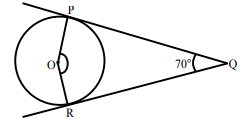Solution:

From the given figure,

∠PQR = 70°

We know that the angle between the two tangents drawn from an external point to a circle is supplementary to the angle subtended by the line segment joining the points of contact at the centre.

∠POR + ∠PQR = 180°

∠POR + 70° = 180°

∠POR = 180° – 70°

∠POR = 110°

Question 5: How many tangents can be drawn on the circle of radius 5 cm from a point lying outside the circle at distance 9 cm from the centre?

Solution:

Given,